# Structure and Formula of Silicates Chemistry Tutorial

## Key Concepts

• The basic building block, the structural unit, of silicate minerals is the SiO44- tetrahedron.
• In the SiO44- tetrahedron each Si atom is covalently bonded to 4 oxygen atoms.
• In the tetrahedron Si has an oxidation state of +4 and O has an oxidation state of -2.

Overall charge on the tetrahedron = +4 + (4 × -2) = -4

• The SiO44- tetrahedron can be represented in different ways.
Some examples of different representations of the same SiO44- tetrahedron are shown below: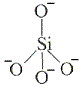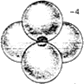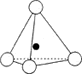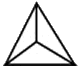• The tetrahedra can link together by sharing corners resulting in Si-O-Si covalent bonds.
A number of different structures are therefore possible.
The table below shows how the tetrahedra join to form rings, chains, bands and sheets:

 discrete "molecules"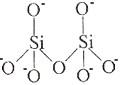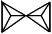discrete rings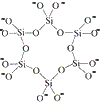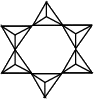infinite single chains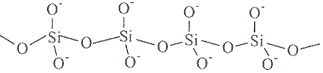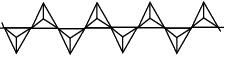infinite double chains (bands)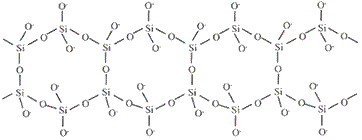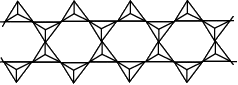infinite sheets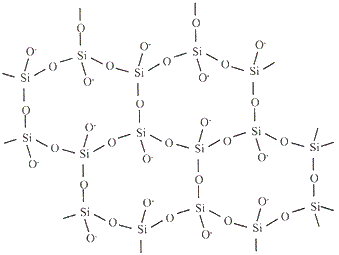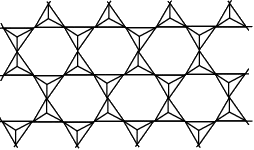infinite three dimensional frameworks (or networks)

• The formula of a silicate anion in a discrete "molecule" is given by the number of silicon atoms and the number of oxygen atoms in the structure:

structure structural formula molecular formula
single tetrahedronSiO44-
charge= +4 + (4×-2)
charge = 4-

2 tetrahedra sharing a cornerSi2O76-
charge = (2 × +4) + (7 × -2)
charge = 6-

3 tetrahedra in a ring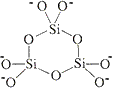Si3O96-
charge = (3 × +4) + (9 × -2)
charge = 6-

6 tetrahedra in a ringSi6O1812-
charge = (6 × +4) + (18 × -2)
charge = 12-

• The formula of a silicate anion in an infinite array can be derived from the repeating unit within the structure:

structure repeating unit shown in red box formula
infinite single chain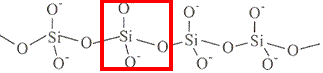SiO32-
charge = +4 + (3 × -2)
charge = -2

infinite double chain
(band)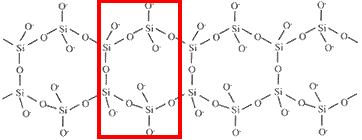Si4O116-
charge = (4 × +4) + (11 × -2)
charge = -6

infinite sheet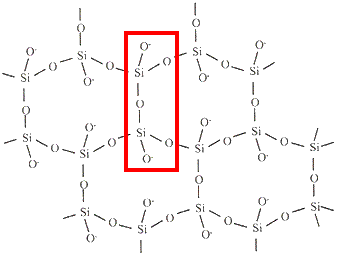Si2O52-
charge = (2 × +4) + (5 × -2)
charge = -2

infinite three dimensional framework (network) SiO2
charge = +4 + (2 × -2)
charge =0

• Cations are required to balance the charge on the silicate tetrahedra (compensating cations).

Some typical compensating cations are:
sodium ions Na+
potassium ions K+
magnesium ions Mg2+
calcium ions Ca2+
aluminium ions Al3+

No ads = no money for us = no free stuff for you!

## Silicate Structure Example : Discrete Tetrahedra

The mineral olivine exists as discrete SiO44- tetrahedra with the charge being balanced by magnesium ions.
We can determine the formula for olivine by balancing the charges on the ions:

1. charge on olivine = charge on silicate anion + charge on cations
The overall charge on olivine = 0
formula of discrete silicate tetrahedron is SiO44-
charge on SiO44- = -4
charge on Mg2+ = +2
2. Let n be the number of Mg2+ required to balance the charge on the silicate ion:
0 = -4 + (n × +2)
+4 = 2n
n = 2
3. formula for olivine is Mg2SiO4

Do you know this?

Play the game now!

## Silicate Structure Example : Single Chain (pyroxene group of silicates)

The mineral enstatite is made up of long single chains of silicate anions with the charge being balanced by magnesium ions.
Electrostatic attraction between the magnesium cations and the silicate anions holds the closely packed chains together.
We can determine the formula for enstatite by balancing the charges on the ions:

1. charge on enstatite = charge on silicate anion + charge on cations
The overall charge on enstatite = 0
formula for silicate anions in long single chains is SiO32-
charge on silicate anions in long single chains = -2
charge on Mg2+ = +2
2. Let n be the number of Mg2+ required to balance the charge on the silicate ion:
0 = -2 + (n × +2)
+2 = 2n
n = 1
3. formula for enstatite is MgSiO3

## Silicate Structure Example : Double Chain (amphibole group of silicates)

The mineral kupfferite is a double chain silicate and has the formula Mg7Si8O22(OH)2.
The repeating unit in kupfferite is the Si4O116- ion, however, in order to avoid fractions for the magnesium cations in the formula the silicate anion formula is doubled to Si8O2212-.
The hydroxide ions, OH-, present in the structure are not bonded to Si atoms within the structural framework, they are co-ordinated around the magnesium cations.
We can check that the mineral kupfferite has no overall charge:
kupfferite charge = (7 × 2+) + (8 × 4+) + (22 × 1-) + (2 × 2-) = 14 + 32 - 44 - 2 = 0

Do you understand this?

Take the test now!

## Silicate Structure Example : Sheet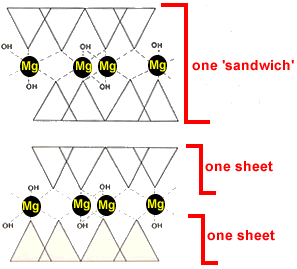The mineral talc is made up of a 'sandwich'of two sheets of tetrahedra with the bases forming the outside of the sandwich as shown in the diagram on the right.
The two layers of sheets are strongly bound together by Mg2+ co-ordinated with two oxygen atoms from each sheet (and two OH-).
The 'sandwich' layers are weakly bound only by weak van der Waals forces, which allows the sandwich layers to slide over each other easily.
Talc is the softest known mineral and is very crumbly which is why it can be used as a lubricant.
The formula for talc is Mg3Si4O10(OH)2.
The repeating silicate anion unit within the structure is Si2O52-, but in order to avoid fractions for the magnesium cations in the formula the silicate anion formula is doubled to Si4O104-.

## Silicate Structure Example : Three Dimensional Networks (Frameworks)

In silica, SiO2, every Si atom is covalently bonded to 4 O atoms, and every O atom is covalently bonded to two Si atoms forming a three dimensional network.
The overall charge on silica is 0 since the oxidation state of silicon (+4) is balanced by the two oxygen atoms with oxidation states of -2 each (2 × -2 = -4).
No cations are required to balance the charge.

Can you apply this?

Join AUS-e-TUTE!

Take the exam now!